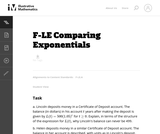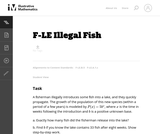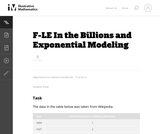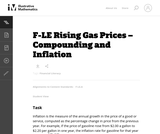Updating search results...

# 6 Results

View
Selected filters:
• MCCRS.Math.Content.HSF-LE.A.1cUnrestricted Use
CC BY
Rating
0.0 stars

This task gives students an opportunity to work with exponential functions in a real world context involving continuously compounded interest. They will study how the base of the exponential function impacts its growth rate and use logarithms to solve exponential equations.

Subject:
Mathematics
Functions
Material Type:
Activity/Lab
Provider:
Illustrative Mathematics
Provider Set:
Illustrative Mathematics
Author:
Illustrative Mathematics
05/01/2012Only Sharing Permitted
CC BY-NC-ND
Rating
0.0 stars

This lesson unit is intended to help teachers assess how well students are able to interpret exponential and linear functions and in particular to identify and help students who have the following difficulties: translating between descriptive, algebraic and tabular data, and graphical representation of the functions; recognizing how, and why, a quantity changes per unit intervale; and to achieve these goals students work on simple and compound interest problems.

Subject:
Algebra
Measurement and Data
Material Type:
Assessment
Lesson Plan
Provider:
Shell Center for Mathematical Education
Provider Set:
Mathematics Assessment Project (MAP)
04/26/2013Only Sharing Permitted
CC BY-NC-ND
Rating
0.0 stars

This lesson unit is intended to help teachers assess how well students are able to: articulate verbally the relationships between variables arising in everyday contexts; translate between everyday situations and sketch graphs of relationships between variables; interpret algebraic functions in terms of the contexts in which they arise; and reflect on the domains of everyday functions and in particular whether they should be discrete or continuous.

Subject:
Functions
Material Type:
Assessment
Lesson Plan
Provider:
Shell Center for Mathematical Education
Provider Set:
Mathematics Assessment Project (MAP)
04/26/2013Unrestricted Use
CC BY
Rating
0.0 stars

This is a direct task suitable for the early stages of learning about exponential functions. Students interpret the relevant parameters in terms of the real-world context and describe exponential growth.

Subject:
Mathematics
Functions
Material Type:
Activity/Lab
Provider:
Illustrative Mathematics
Provider Set:
Illustrative Mathematics
Author:
Illustrative Mathematics
05/01/2012Unrestricted Use
CC BY
Rating
0,0 stars

This problem provides an opportunity to experiment with modeling real data. Populations are often modeled with exponential functions and in this particular case we see that, over the last 200 years, the rate of population growth accelerated rapidly, reaching a peak a little after the middle of the 20th century and now it is slowing down.

الموضوع:
الرياضيات
وظائف
نوع المادة:
Activity/Lab
Provider:
Illustrative Mathematics
Provider Set:
Illustrative Mathematics
المؤلف:
Illustrative Mathematics
05/01/2012Unrestricted Use
CC BY
Rating
0.0 stars

The purpose of this task is to give students an opportunity to explore various aspects of exponential models (e.g., distinguishing between constant absolute growth and constant relative growth, solving equations using logarithms, applying compound interest formulas) in the context of a real world problem with ties to developing financial literacy skills.

Subject:
Mathematics
Functions
Material Type:
Activity/Lab
Provider:
Illustrative Mathematics
Provider Set:
Illustrative Mathematics
Author:
Illustrative Mathematics